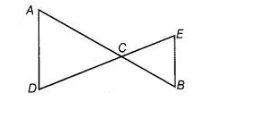`
Question:

In the adjoining figure, we have AC = DC and CB = CE. Show that AB = DE.Solution:

Given, AC = DC …(i)

and C6 = CE …(ii)

According to Euclid’s axiom, if equals are added to equals, then wholes are also equal.

So, on adding Eqs.(i) and (ii), we get

AC + CB = DC + CE

=> AB = DE